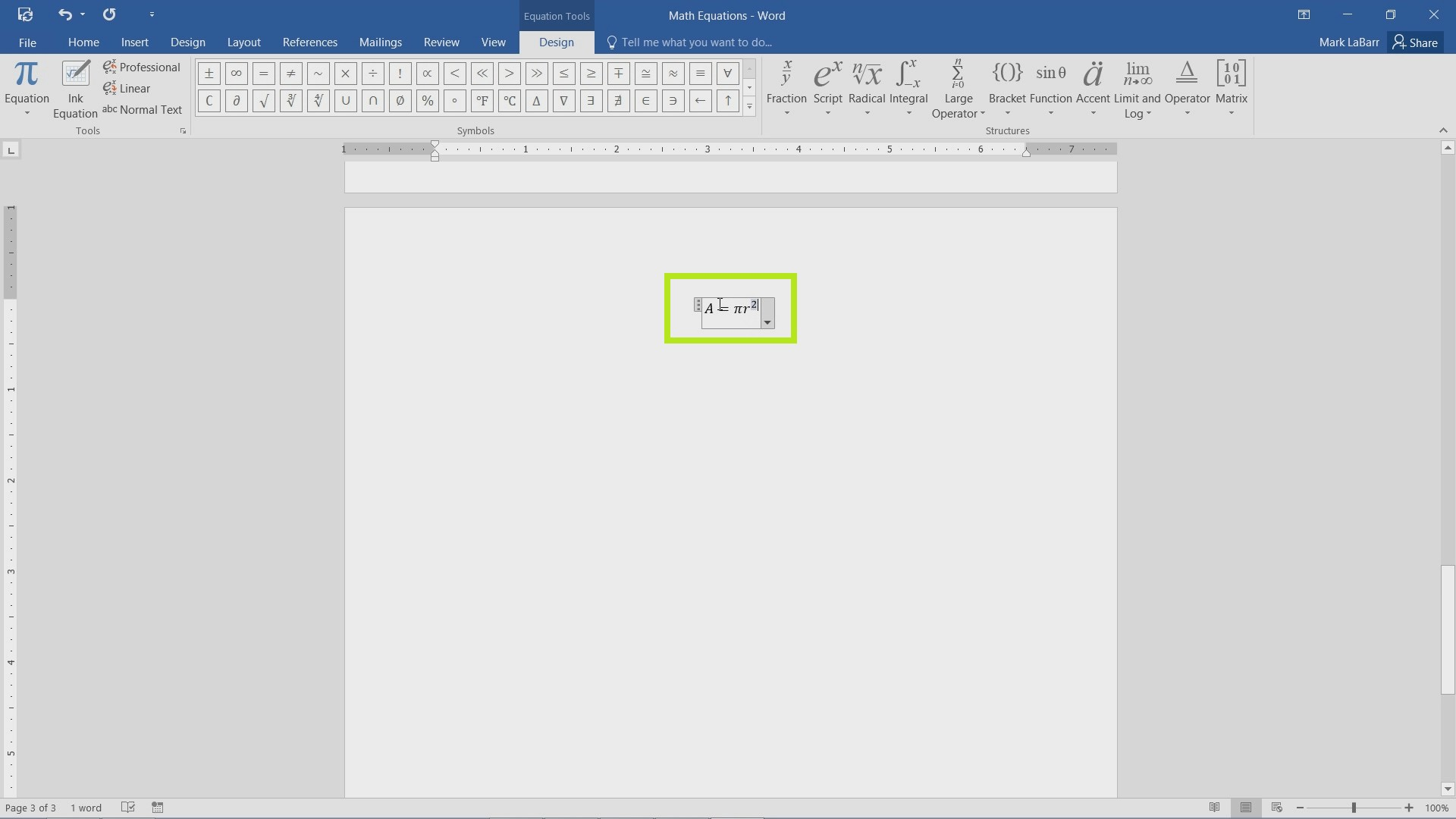## How to Create Equations in Word 2016

In this tutorial, we’re going to show you how to create equations in Word 2016.

To get started, go under the Insert tab, click either the Equations button to insert a new equation or the drop-down arrow for more options.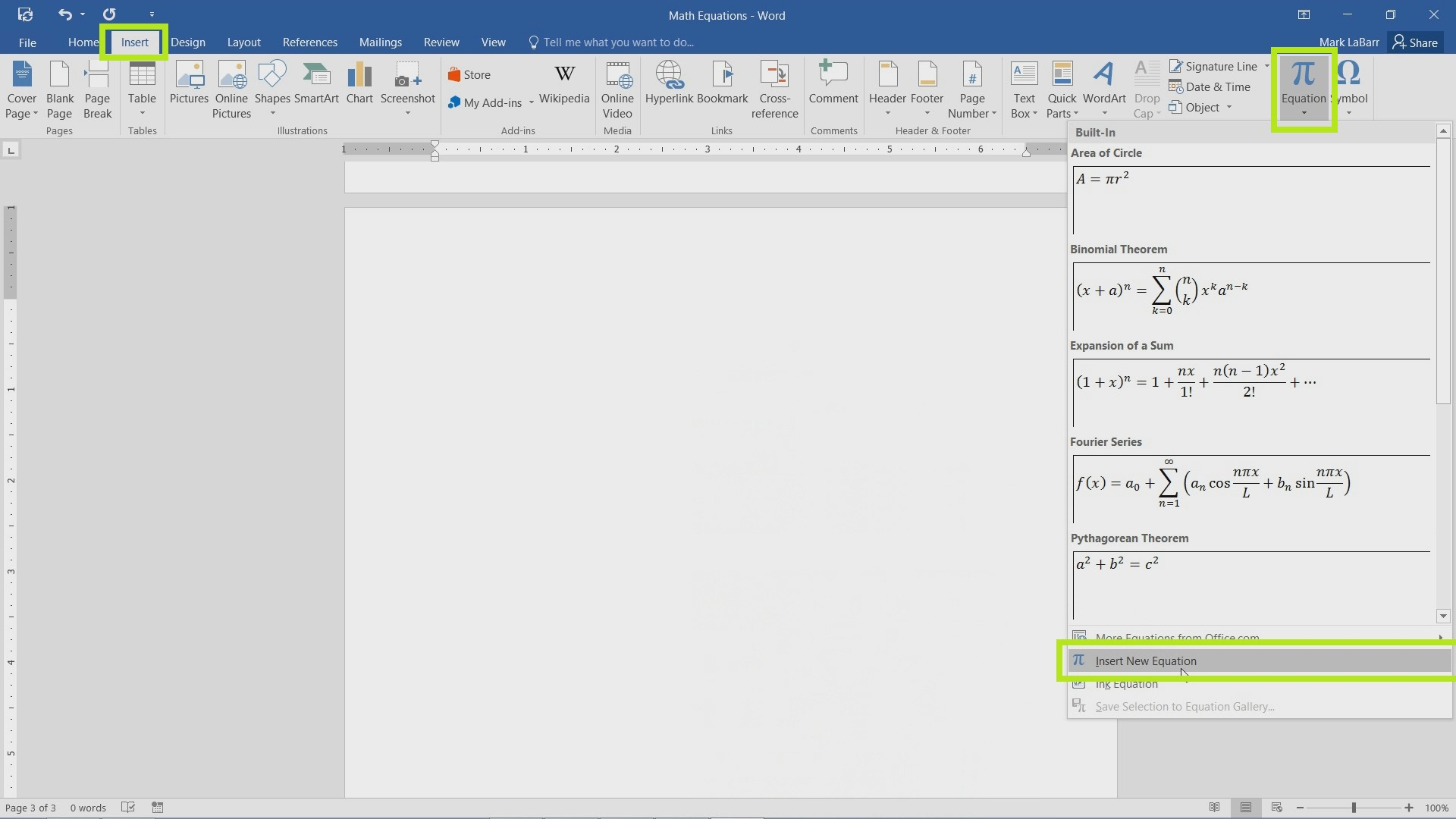You can also Ink an equation from this menu. Inking equations is a new feature that allows you to draw up an equation with a cursor. This feature is very helpful if a drawing tablet is handy.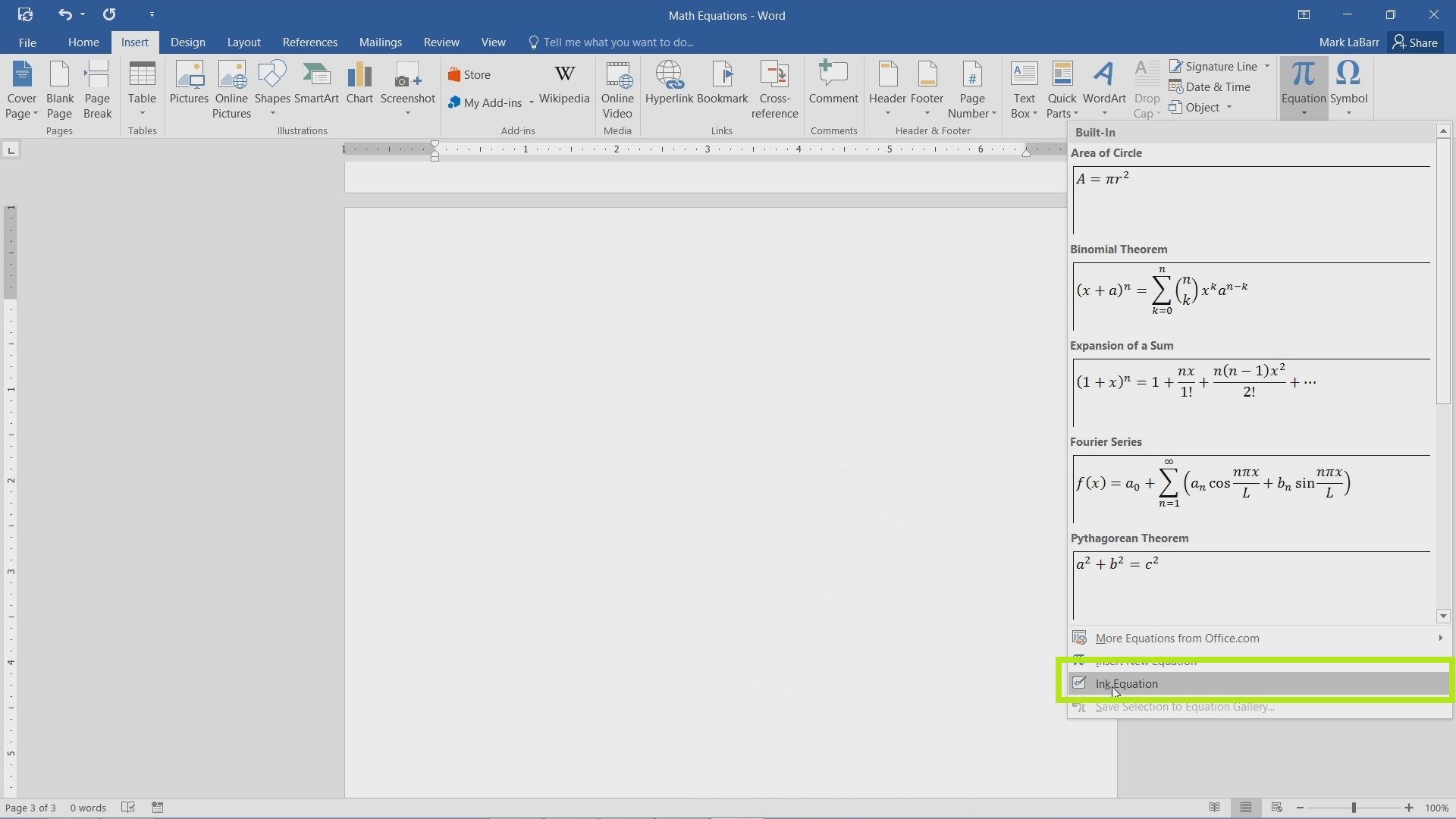An interface as shown below should show up after inserting a new equation.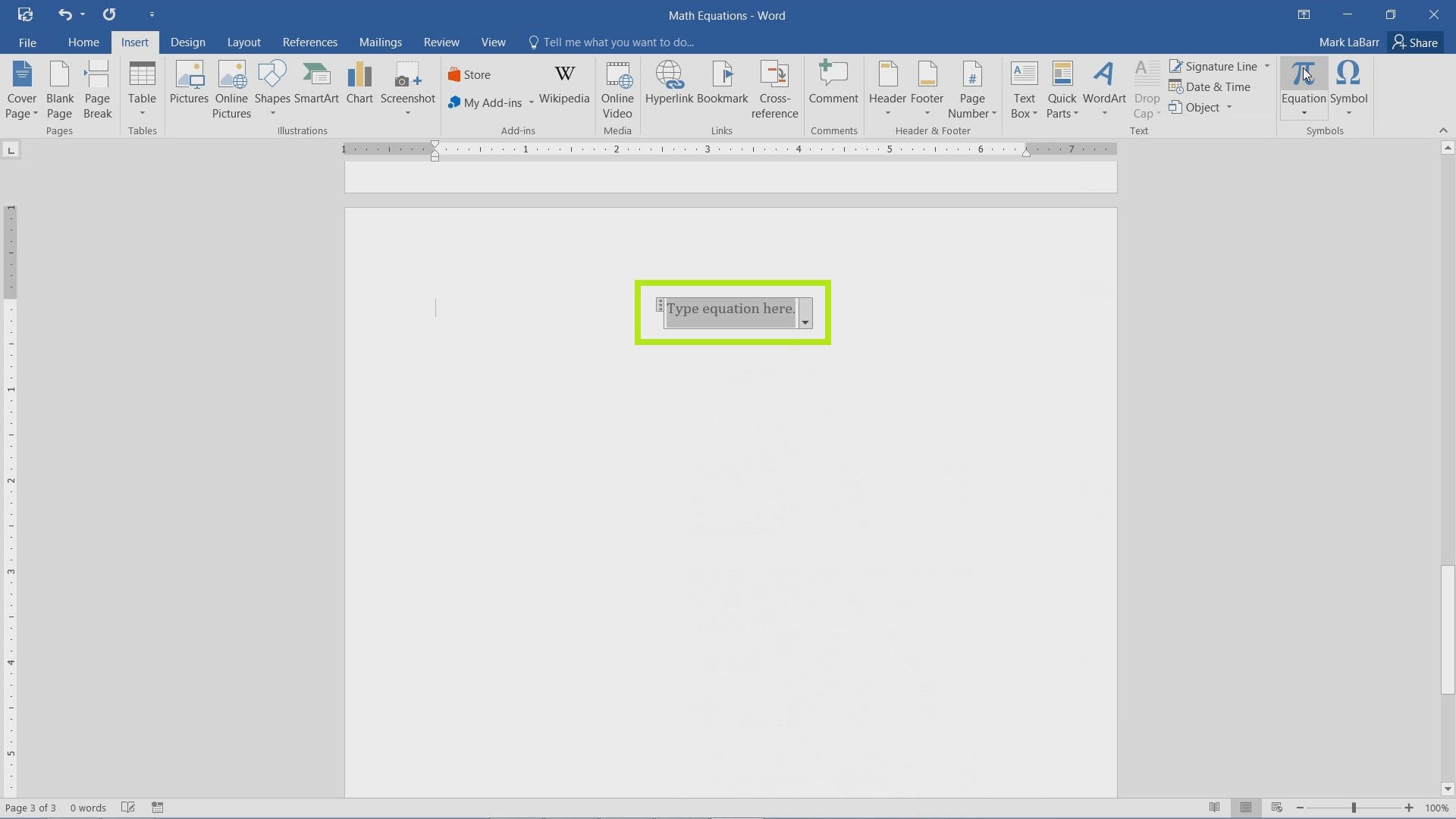You can also Ink an equation for a newly created equation if you decide to do so.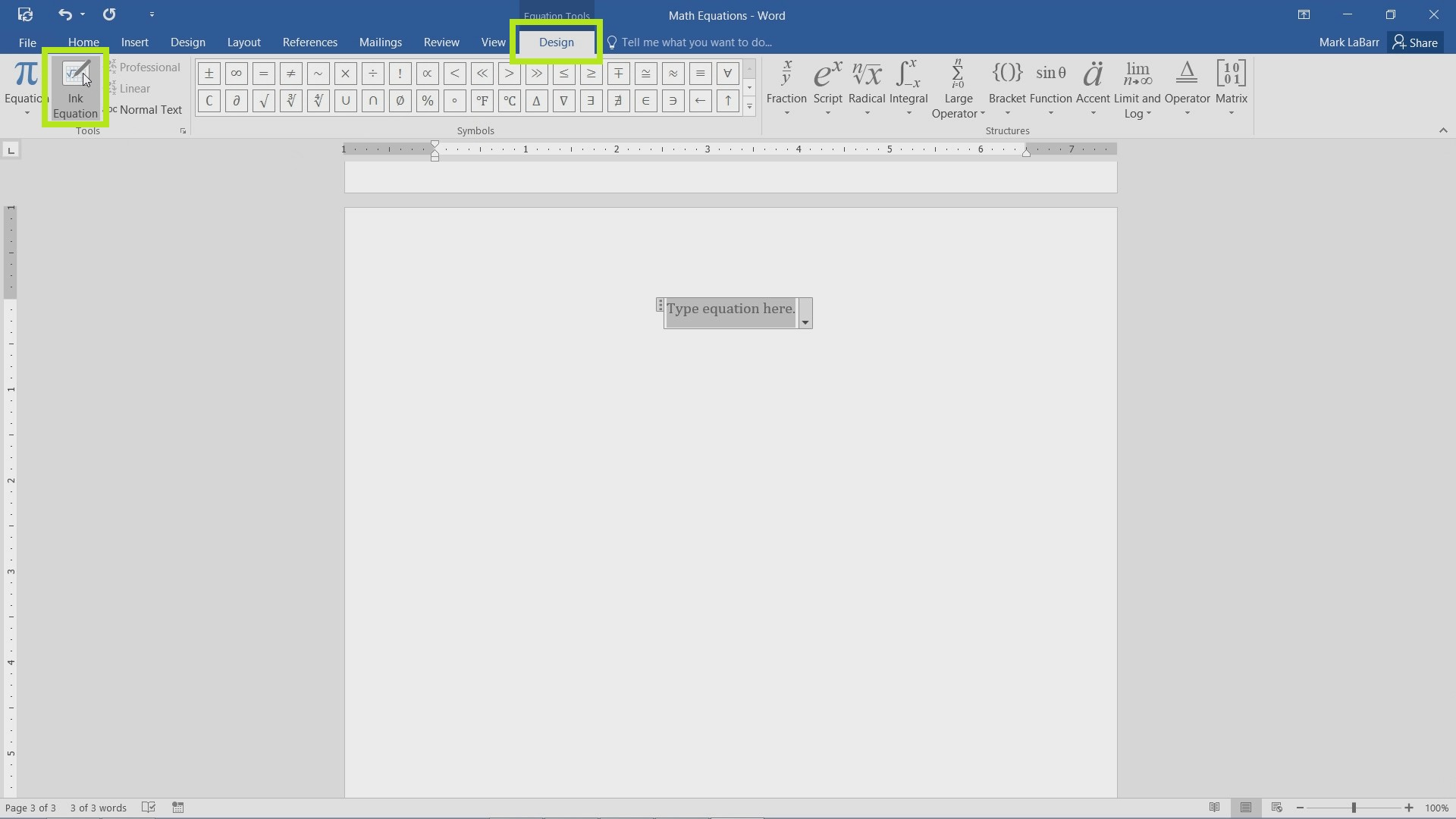This equation was done using a mouse. As you can see, Word 2016 correctly interpreted it.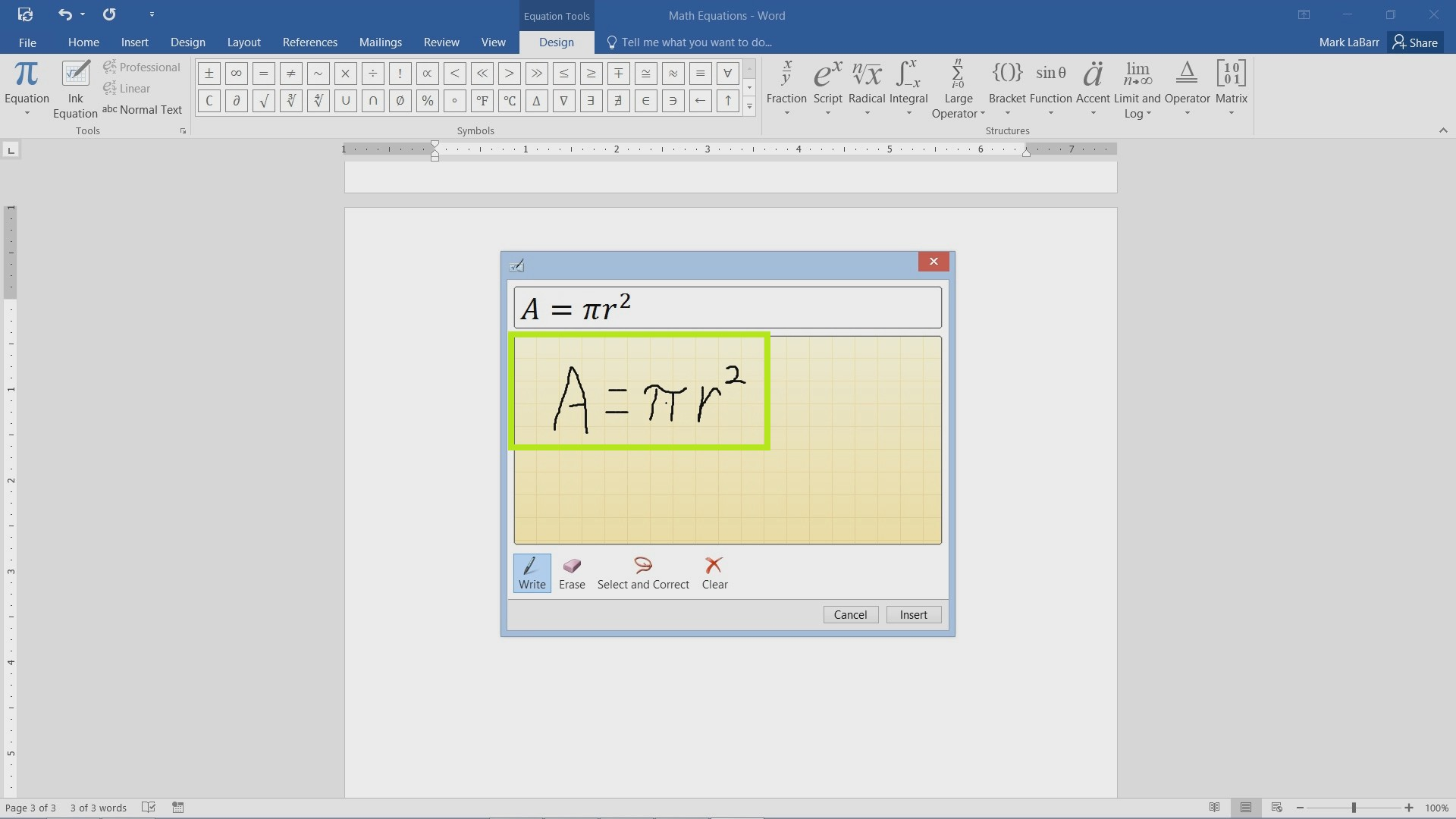Here the finished equation is shown.